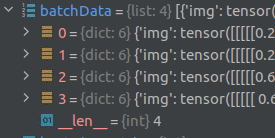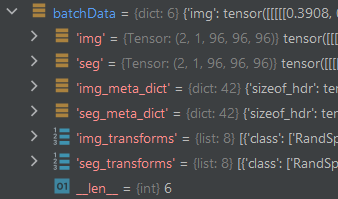# Incorrect shape of data from DataLoader

DataLoader is returning wrong shape when using BTCV dataset.

This is my dataset, which contains pairs of CT image and segmentation label in NIFTI format. Result shape of TRAIN_PAIR_PATHS is List of dicts

``````file_list = os.listdir(f"{DATASET_PATH}/img/")
val_files = set(random.sample(file_list, NUMBER_OF_VAL_FILES))

TRAIN_PAIR_PATHS = [
{"img": f"{DATASET_PATH}img/{f}", "seg": f"{DATASET_PATH}label/{f.replace('img', 'label')}"}
for f in set(file_list) - val_files
]
``````

``````trainLoader = DataLoader(
batch_size=2,
dataset=CacheDataset(data=TRAIN_PAIR_PATHS, transform=TRAINING_PRE_TRANSFORM, cache_rate=1.), shuffle=True
)
``````

I expect that data can be accessed like this via keys:

`````` print(batchData['img'].shape)
print(batchData['seg'].shape)
``````

but dataloader is returning me this shape when using standard loop like this:

``````for batchData in trainLoader:
another code
``````Expected outputCan you please share your CacheDataset class and an example of the wrong shape that you get vs the expected shape?

Hi CacheDataset is provided by MONAI framework (Data — MONAI 1.1.0 Documentation) and I also attached output of debugger …as you can see wrong shape is list of dicts. I also added expected shape. THanks

The `DataLoader` should be able to consume `dict`s as seen here:

``````class MyDataset(Dataset):
def __init__(self):
self.data = torch.arange(10)
self.target = torch.arange(10, 20)

def __len__(self):
return len(self.data)

def __getitem__(self, index):
x = self.data[index]
y = self.target[index]
return {"img": x, "target": y}

dataset = MyDataset()
print(dataset)
# {'img': tensor(0), 'target': tensor(10)}

print(batch)
print(batch["img"].shape)

# {'img': tensor([0, 1, 2, 3, 4]), 'target': tensor([10, 11, 12, 13, 14])}
# torch.Size()
# {'img': tensor([5, 6, 7, 8, 9]), 'target': tensor([15, 16, 17, 18, 19])}
# torch.Size()
``````

but it’s unclear how you are setting up your dataset and how the samples are created.

Since the input / output format seems unusual wouldn’t it be a suitable case for using custom-made collate_fn?

Maybe a custom `collate_fn` could help as I don’t know why the original code doesn’t work.
My code snippet shows that `dict`s are acceptable and won’t create the mentioned output.

Isn’t it because the batch contains elements that are not tensors (dicts and list) that pytorch unable to batch? in your last code snippet you are returning a dict but its values are tensors so it is clear how to batch across samples. As I understand the question we will have:

``````   def __getitem__(self, index):
x = img, img_meta_dict, img_transforms # tensor, dict, list
y = seg, seg_meta_dict, seg_transforms # tensor, dict, list
return {"img": x, "target": y}
``````

@David_Hresko I think you can change the get_item for something as follow:

``````   def __getitem__(self, index):
img_transforms = Compose(img_transforms)
x = img_transforms(img)
seg_transforms = Compose(seg_transforms)
y = seg_transforms(img)

return {"img": x, "target": y, "img_meta": img_meta_dict, "seg_meta": seg_meta_dict}
``````

and:

``````for (imgs, targets, imgs_meta, targets_meta) in train_loader:
your_code
``````

then the imgs and targets will have the expected shapes